## RD Sharma Class 8 Solutions Chapter 8 Division of Algebraic Expressions Ex 8.4

These Solutions are part of RD Sharma Class 8 Solutions. Here we have given RD Sharma Class 8 Solutions Chapter 8 Division of Algebraic Expressions Ex 8.4

Other Exercises

Question 1.
5x3 – 15x2 + 25x by 5x
Solution:Question 2.
4z3 + 6z2-zby $$\frac { -1 }{ 2 }$$ z
Solution: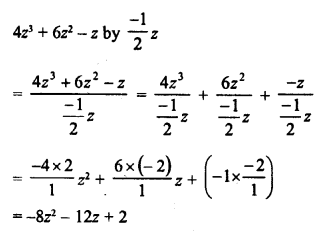Question 3.
9x2y – 6xy + 12xy2 by $$\frac { -3 }{ 2 }$$ xy
Solution: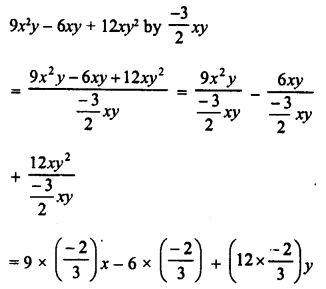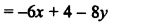Question 4.
3x2y2 + 2x2y + 15xy by 3xy
Solution: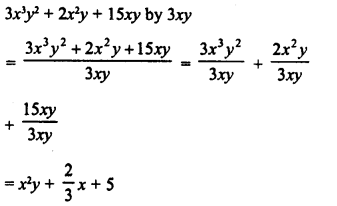Question 5.
x2 + 7x + 12 by x + 4
Solution:Question 6.
4y4 + 3y + $$\frac { -1 }{ 2 }$$ by 2y + 1
Solution: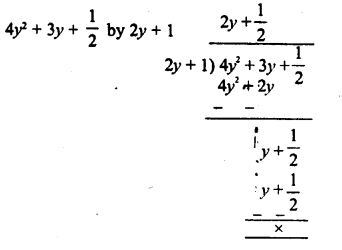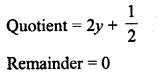Question 7.
3x3 + 4x2 + 5x + 18 by x + 2
Solution:Question 8.
14x2 – 53x + 45 by 7a – 9
Solution: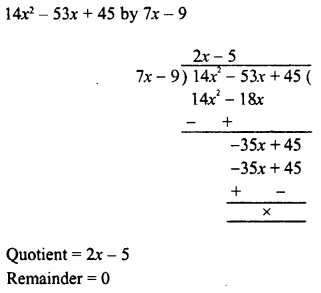Question 9.
-21 + 71x – 31x2 – 24ax3 by 3 – 8ax
Solution: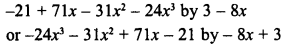Question 10.
3y4 – 3y3 – 4y2 – 4y by y2 – 2y
Solution:Question 11.
2y5 + 10y4 + 6y3 + y2 + 5y + 3 by 2y3 + 1
Solution: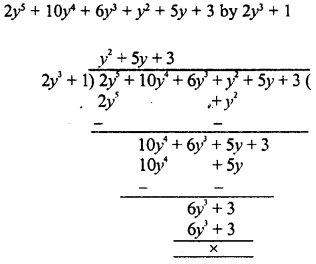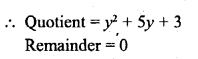Question 12.
x4 – 2x3 + 2x2 + x + 4 by x2 + x + 1
Solution: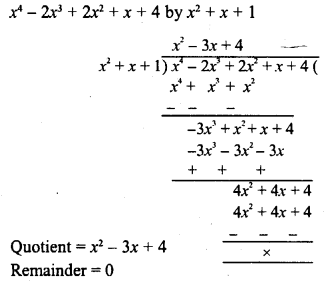Question 13.
m3 – 14m2 + 37m – 26 by m2 – 12m + 13
Solution:Question 14.
x4 + x2 + 1 by x2 + x + 1
Solution: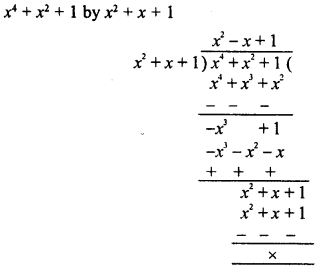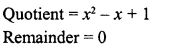Question 15.
x5 + x4 + x3+x2 + x+ 1 by x3 + 1
Solution:Divide each of the following and find the quotient and remainder :

Question 16.
14x3 – 5x2 + 9x -1 by 2x – 1
Solution: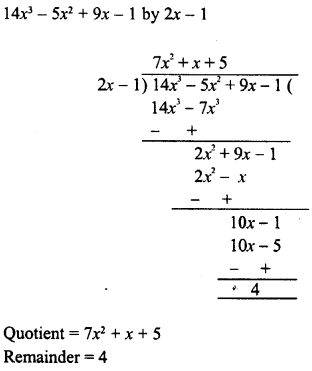Question 17.
6x3 – x2 – 10x – 3 by 2x – 3
Solution: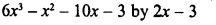Question 18.
6x3+ 11x2 – 39x – 65 by 3x2 + 13x + 13
Solution: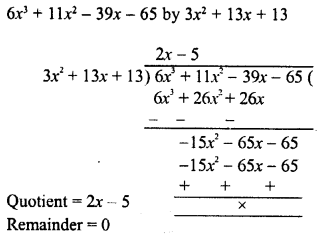Question 19.
30a4 + 11a3-82a2– 12a + 48 by 3a2 + 2a- 4
Solution: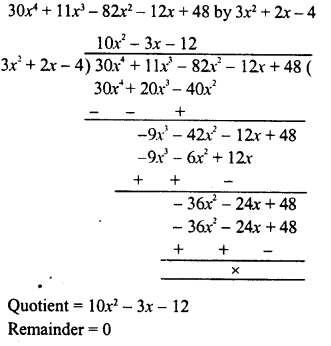Question 20.
9x4 – 4x2 + 4 by 3x2 – 4x + 2
Solution: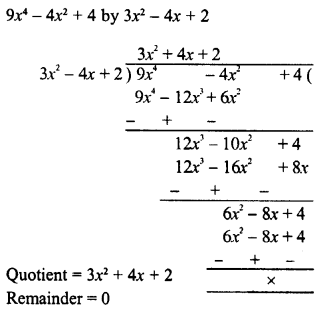Question 21.
Verify division algorithm i.e., Dividend = Divisor * Quotient + Remainder, in each of the following. Also, write the quotient and remainder :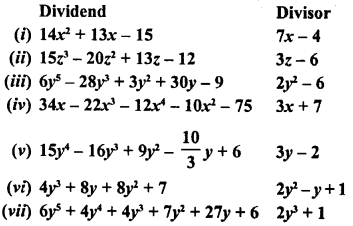Solution: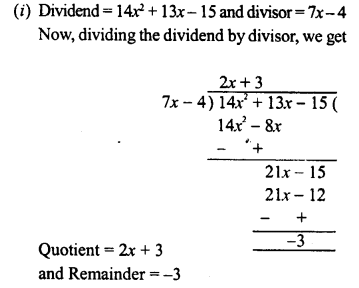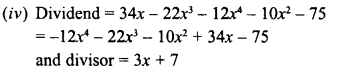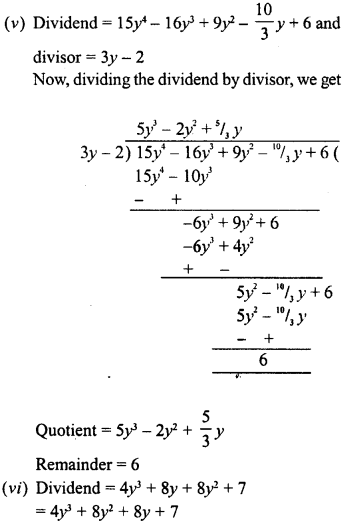Question 22.
Divide 15y4 + 16y3 + $$\frac { 10 }{ 3 }$$ y – 9y2 – 6 by 3y – 2
Write down the co-efficients of the terms in the quotient.
Solution: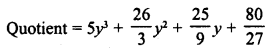Question 23.
Using division of polynomials state whether.
(i) x + 6 is a factor of x2 – x – 42
(ii) 4x – 1 is a factor of 4x2 – 13x – 12
(iii) 2y – 5 is a factor of 4y4 – 10y3 – 10y2 + 30y -15
(iv) 3y + 5 is a factor of 6y5 + 15y + 16y + 4y+ 10y – 35
(v) z2 + 3 is a factor of z5– 9z
(vi) 2x2 – x + 3 is a factor of 60x5-x4 + 4x3 – 5x2 -x- 15
Solution: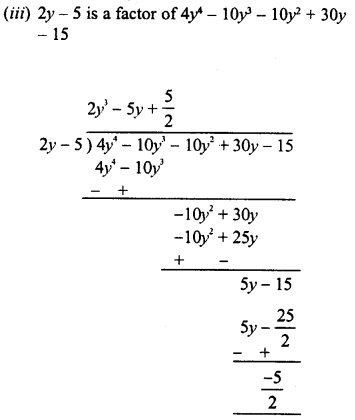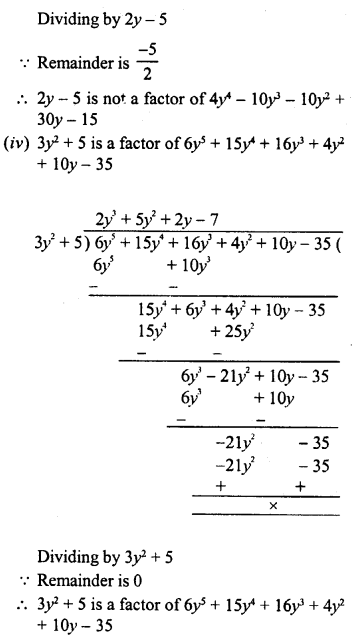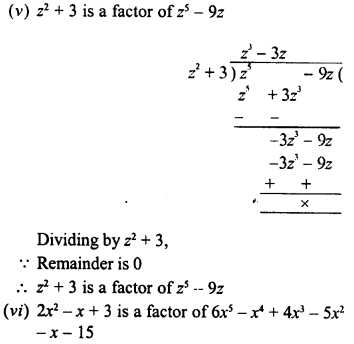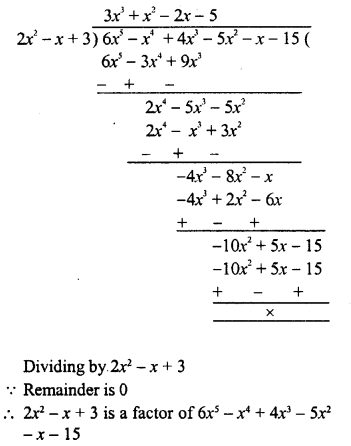Question 24.
Find the value of ‘a’, if x + 2 is a factor of 4x4 + 2x3 – 3x2 + 8x + 5a.
Solution: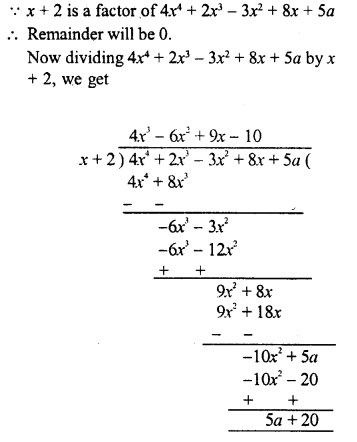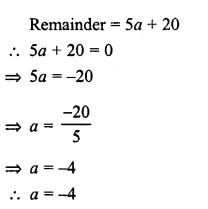Question 25.
What must be added to x4 + 2x3 — 2x2 + x – 1 so that the resulting polynomial is exactly divisible by x2 + 2x – 3.
Solution: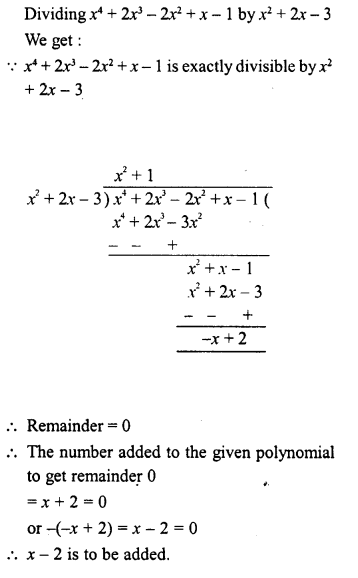Hope given RD Sharma Class 8 Solutions Chapter 8 Division of Algebraic Expressions Ex 8.4 are helpful to complete your math homework.

If you have any doubts, please comment below. Learn Insta try to provide online math tutoring for you.